Mobile QR Code1. (The authors are with the State Key Laboratory of Electronic Thin Films and Integrated Devices, University of Electronic Science and Technology of China, Chengdu 610054, China )

High-k dielectric, power MOSFET, silicon-on-insulator

## I. INTRODUCTION

The lateral double-diffused MOSFET (LDMOS) has a wide application in smart power integrated circuits, and the silicon-on-insulator technology is attractive for its inherent characteristics of the effective isolation and lower leakage current (1)-(3). For a semiconductor device, a low conduction loss and a high breakdown voltage (BV) are always crucial design targets. However, for an LDMOS, there is a compromise relationship between them, which has a close affinity with the surface electric filed along the drift region. A uniform distribution of the surface electric field yields a high BV, which is thus desired (4),(5). A modulation on electric fluxes, by a field plate, can enhance the strength of local electric field for improving its distribution (6)-(8), and it is an effective method to improve the compromise relationship between the BV and the specific on-resistance ($R_{\mathrm{on,sp}}$).

In this paper, based on the idea of modulation on electric fluxes, an SOI LDMOS with three-section high-k dielectrics placed over the drift region is proposed and investigated by TCAD simulations. Local electric field strength is enhanced in the drift region, which achieves a larger average strength of the electric field, with a higher BV. The $R_{\mathrm{on,sp}}$ is decreased in the meanwhile, since the high-k dielectric provides a preferable path for electric fluxes flowing through (9),(10), and the dose of the drift region is possible to be increased.

## II. DEVICE STRUCTURE AND MECHANISM

Fig. 1. Device structures of (a) conventional SOI LDMOS, (b) proposed SOI LDMOS with variable-k dielectric.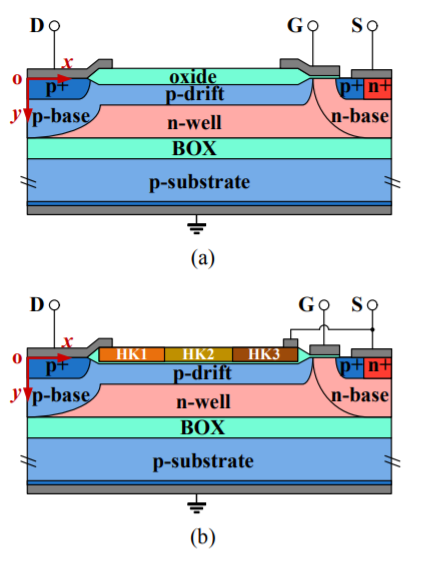Device structures of a conventional LDMOS (11) and the proposed LDMOS are schematically shown in Fig. 1. As shown in the figure, the proposed device features a three-section variable-k dielectric placed over the drift region. The dielectric constants are increased from the drain side to the source side. Based on the theory of electromagnetics, the electric flux is continuous. As for the specific case of the proposed device, the electric fluxes flowing into the dielectric ($D_{x-i n}$) are equal to the electric fluxes flowing out of the dielectric ($D_{x-out}$ + $D_{y-out}$), where $D_{y-out}$ represents extra electric fluxes introduced into the drift region by high-k dielectrics. $D_{y-out}$ is caused by the potential difference between the high-k dielectric and the surface of the drift region. From the source side to the drain side, namely form the high-potential side to the low-potential side, the dielectric constants for high-k dielectrics need to be accordingly decreased, to math this condition, for achieving a more uniform distribution of the electric field and a higher BV.

The advantage of the utilization of high-k dielectrics is not only an enhancement on the BV but also a decrease in the $R_{\mathrm{on,sp}}$. A medium with large permittivity has a nature of highly polarization under the force by electric field, which means most electric field lines prefer to flow through a high-k dielectric compared with a low-k dielectric. Fig. 2(b) and (c) schematically illustrate the influence of a high-k dielectric on the electric fluxes. In the drift region, the electric fluxes caused by the ionized impurity ions would like to flow through the high-k dielectric rather than the silicon. In other words, the distribution of the electric field is barely affected by an appropriate increment in the dose of the drift region, since the high-k dielectric provides a preferable path for electric fluxes flowing through.

Fig. 2. Mechanism of proposed LDMOS. (a) Relationship of electric field in two different mediums. (b) Schematic electric flux lines in a conventional device. (c) Schematic electric flux lines under the influence by high-k dielectric.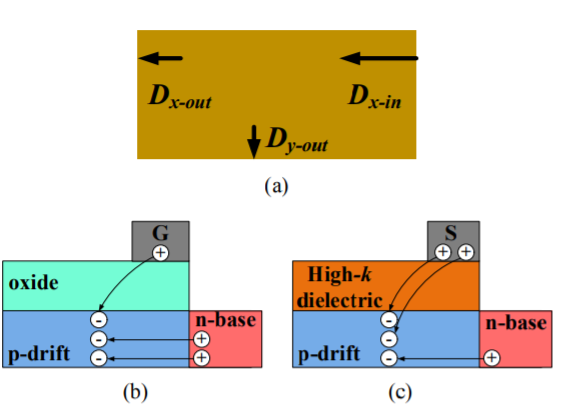## III. RESULTS AND DISCUSSION

The proposed device is investigated by TCAD simulator Synopsys Sentaurus. In the simulation, the thicknesses of the BOX and the SOI layer on it are 3 ${\mu}$m and 2.5 ${\mu}$m, respectively. The drift region length is 20 ${\mu}$m. The doping concentration of the n-well is 2.5 ${\times}$ 10$^{16}$ cm$^{-3}$. The dose of the p-drift region is 4.4 ${\times}$ 10$^{12}$ cm$^{-2}$ for the previous structure, and it is 5.2 ${\times}$10$^{12}$ cm$^{-2}$ for the proposed device. The dielectric constants for HK1, HK2 and HK3 are 20, 100 and 200, respectively. Referring to the reported researches (12)-(14), these high-k dielectrics could be HfO$_{2}$, SrTiO$_{3}$, Ba$_{\mathrm{1-x}}$Sr$_{\mathrm{x}}$TiO$_{3}$, et al.

Fig. 3 shows the distribution of equipotential contours for the conventional LDMOS and the proposed device. In the conventional LDMOS, equipotential contours concentrate at both ends of the drift region, which indicates that there are high electric field strengths at these places. In comparison, a more uniform distribution of equipotential contours is achieved in the proposed device with the help of high-k dielectrics, which also indicates a more uniform distribution of electric field. Fig. 4 compares the flowing directions of electric flux for the conventional LDMOS and the proposed device at their respective BVs. It can be seen in the figure that more electric fluxes prefer to flow, upwards, into high-k dielectric, which directly verifies that the high-k dielectrics have an effect of modulating the distribution of the electric fluxes.

Fig. 3. Equipotential contours at BV for (a) conventional LDMOS and (b) proposed LDMOS.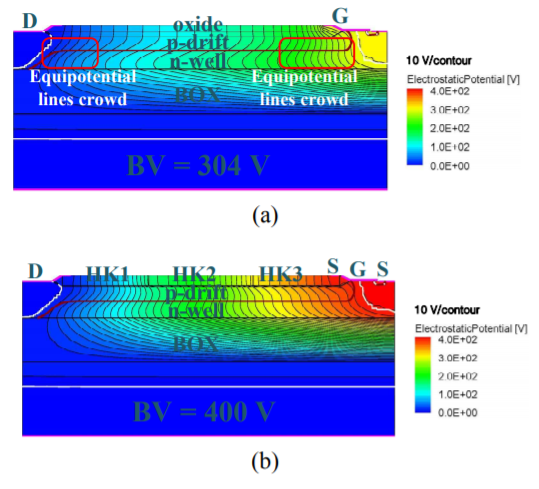Fig. 4. Streams of electric flux at BV for (a) conventional LDMOS and (b) proposed LDMOS.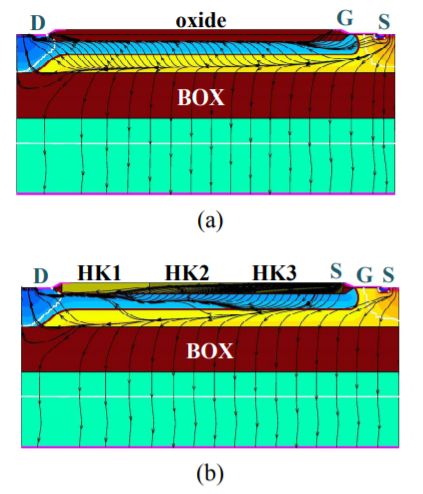The profile of electric field ($E$) along the surface of the drift region in the proposed LDMOS is shown in Fig. 5, including values of the $x$-component ($E_{x}$) and the $y$-component ($E_{y}$) of $E$, corresponding to the direction shown in Fig. 1. The comparison with the conventional device, depicted in dashed lines, is also shown in the same figure. Due to the variation in the permittivity of high-k dielectric, the electric field forms steps at the interfaces of HK1/HK2 and HK2/HK3, where the permittivity is not continuous. The introduction of extra electric fluxes by high-k dielectrics optimizes the distribution of electric field, and the average electric field strength and the BV of the proposed LDMOS are finally enhanced.

The high-k dielectric not only enhances the BV but also provides a preferable path for electric fluxes flowing through (as shown in Fig. 4(b)). An appropriate increment in the dose of the drift region is thus possible to reduce the $R_{\mathrm{on,sp}}$. In the meanwhile, the BV is not sacrificed, as the reason discussed in Section II. Simulation results indicate that, at the gate-source voltage of -15 V, the BV increases from 304 to 400 V by 31%, and the $R_{\mathrm{on,sp}}$ decrease from 71.8 to 62.3 mΩ·cm$^{2}$ by 13%. Judging by BV$^{2}$/$R_{\mathrm{on,sp}}$, the figure of merit (FOM) increases approximately 2 times.

Fig. 6. Dependence of the breakdown voltage on key parameters. (a) The BV as a function of the permittivity of the high-k dielectric. (b) Influence of the dose of the drift region on the BV.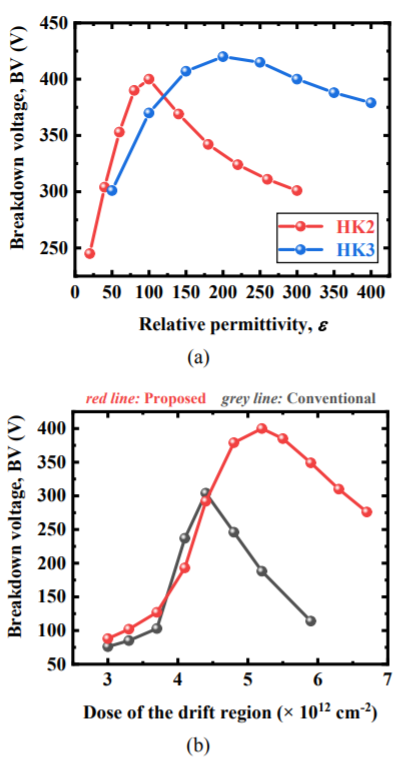Fig. 6 presents the relationship between the BV and the dielectric constants of high-k dielectrics, and the effect of the dose of the drift region on the BV. In Fig. 6(a), high-k dielectrics with different dielectric constants would affect the distribution of electric field, since different amounts of electric fluxes are introduced into the surface of the drift region. The BV increases first and then decreases with the increase in the permittivity of HK2, under the condition that the dielectric constants for the other two high-k dielectrics are keeping constants. The BV has a similar variation, with an increase in the permittivity of HK3. In Fig. 6(b), compared with the conventional device, the deviation on the dose of the drift region does not greatly affect the BV in the proposed device, and it is possible for the proposed device to have a larger process window.

Fig. 7. Capacitances of LDMOS versus source to drain voltages.Fig. 7 shows the capacitances between the gate G and the other two terminals, the source S and the drain D. Due to a large permittivity of the high-k dielectric, the capacitances between the gate and the drain ($C_{GD}$) and between the gate and the source ($C_{GS}$) both increase approximately one order of magnitude. An obvious increment in the gate charge is thus required for the switching process, as shown in Fig. 8.

Fig. 8. Comparison of gate charge between the conventional and the proposed LDMOS.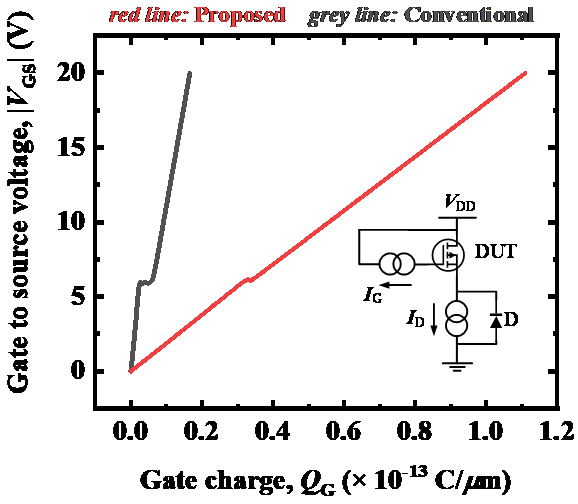Fig. 9. Switching performance. (a) Turn-on process. (b) Turn-off process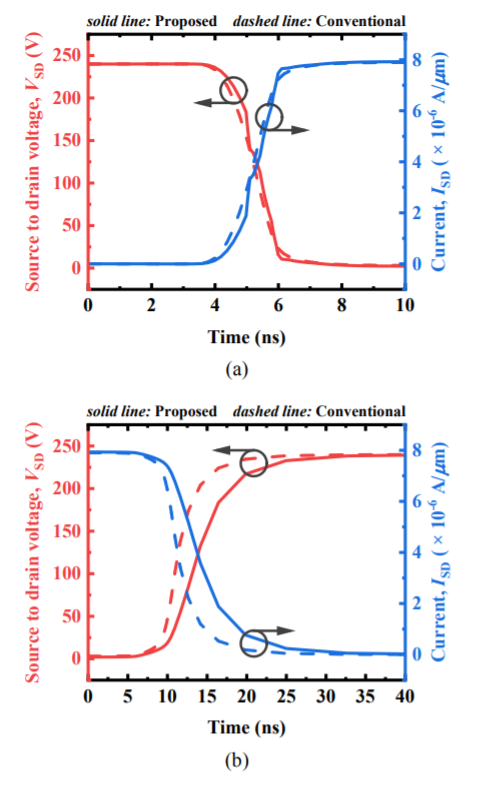Fig. 9 compares the switching performances for the conventional LDMOS and the proposed device. Due to the increased capacitances, the turn-on process is delayed slightly. For the turn-off process, the increment in the dose of the drift region in the proposed device makes the turn-off time larger than that of the conventional device, since it takes more time to drain away these increased carriers.

Fig. 10. Output characteristics of the proposed LDMOS at different temperatures.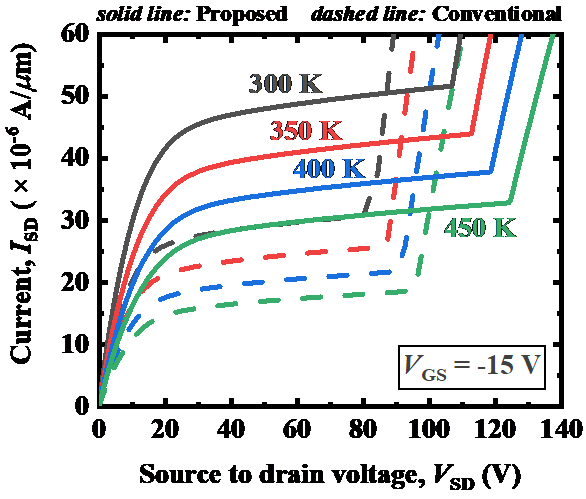Fig. 10 illustrates the DC output characteristics of the proposed device at different temperatures. As the current, flowing through an LDMOS, is of negative temperature coefficient, a higher temperature leads a smaller current. Thermal dynamic simulations of $I_{SD}$-$V_{SD}$ for the conventional LDMOS and the proposed device are compared in Fig. 11. Compared with the conventional LDMOS, due to a large current capability, much heat is generated during the conduction in the proposed device, and the current degradation is slightly heavy for the self-heating effect, which is characterized by a large slope of the $I_{SD}$-$V_{SD}$ curve.

Fig. 11. Thermal dynamic simulation of \textit{I}$_{\mathrm{SD}}$-\textit{V}$_{\mathrm{SD}}$.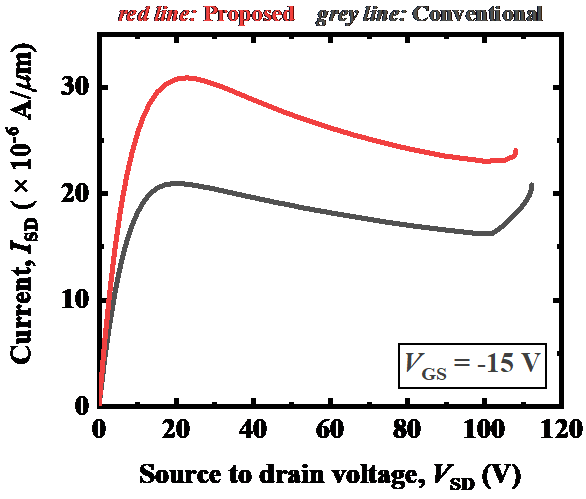Fig. 12. Fabrication process for variable-k dielectric layer.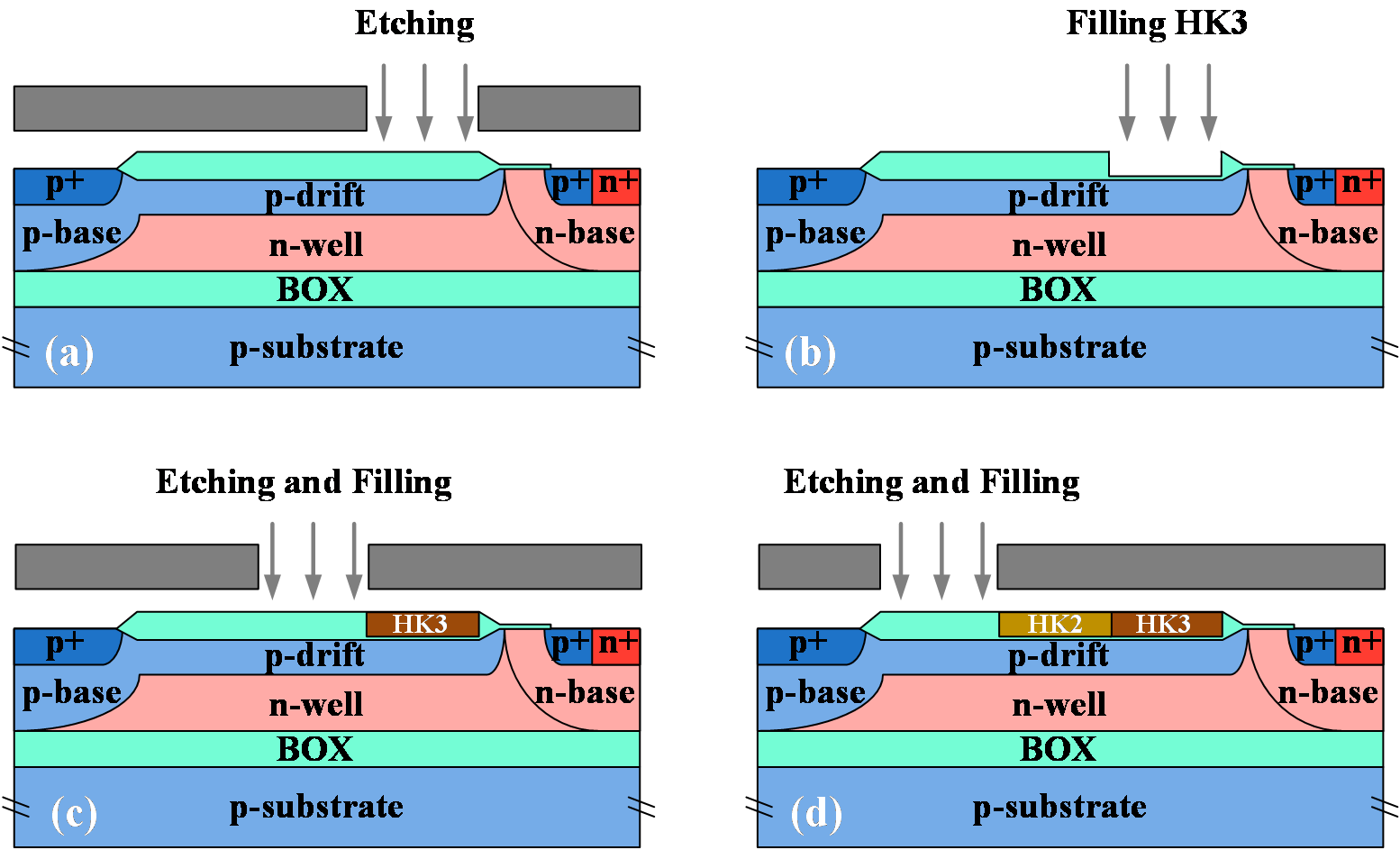Fig. 13. Influence of interface charges on the breakdown voltage.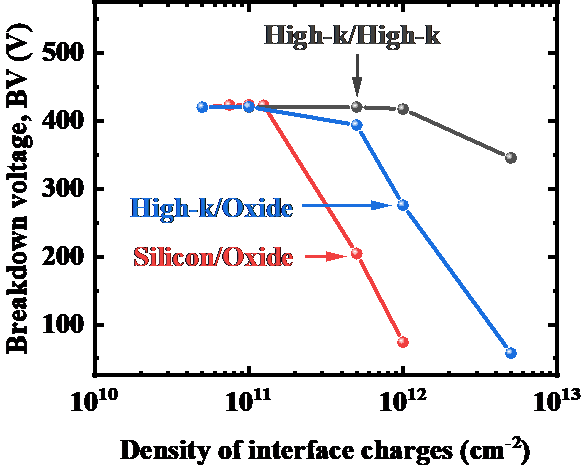Fig. 12 depicts a possible fabrication process for the variable-k dielectric layer over the drift region. The other processes are same to those of the conventional device, which is not detailed in this paper. The oxide over the drift region is first etched to form a trench, and then filled with a high-k dielectric. The high-k dielectric can be fabricated by a method called as “Sol-Gel” (15),(16) that is compatible with IC manufacturing process.

In the actual fabrication, different process would affect the amount of interface charges, which has a close relationship with device characteristics, especially the breakdown voltage. These charges exist at the interfaces of different materials. Fig. 13 shows the influence on the breakdown voltage by interface charges. When the density of interface charges is small, e.g. from 5 ${\times}$ 10$^{10}$ to 1 ${\times}$10$^{11}$ cm$^{-2}$, the breakdown voltage almost remains constant. When the density of interface charges is larger, the electric fluxes caused by these charges are stronger enough to affect the distribution of the electric field, and the breakdown voltage is thus reduced.

In an application, although it is possible to fabricate materials with demanded dielectric constants (10),(12)-(14), for a certain specific case, there may be a limitation or a difficulty to obtain three different demanded dielectrics together. For addressing this problem, an alternative structure is proposed in this paper as an alternative plan, which is schematically illustrated in Fig. 14(a). Compared with Fig. 1(b), the feature of the alternative structure is that HK2 is replaced by HK1 and HK3, which forms an overlap region. Fig. 14(b) shows the influence of the heights of HK1 and HK3 on the BV, and the alternative structure has the highest BV of 392 V, which is almost the same with the structure in Fig. 1(b).

Fig. 14. The second proposed LDMOS. (a) Schematic device structure. (b) Influence of key parameter on the breakdown voltage.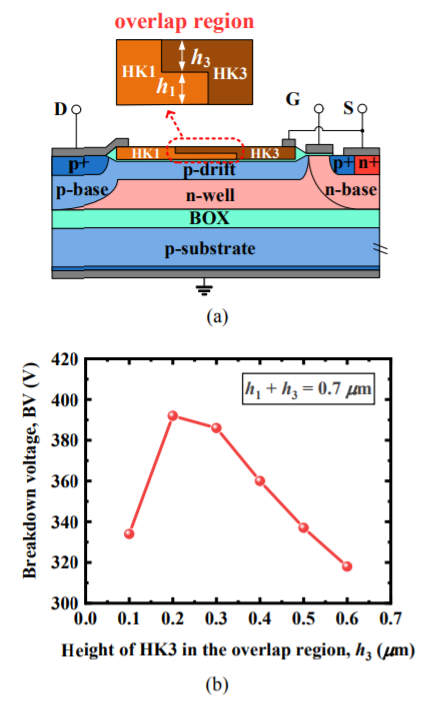## IV. CONCLUSIONS

In this paper, an improved SOI LDMOS with high-k dielectrics is proposed and investigated by TCAD simulations. Beneficial from high-k dielectrics, the BV has been enhanced by the introduction of extra electric fluxes in the drift region to optimize the distribution of the surface electric field. Moreover, the $R_{\mathrm{on,sp}}$ has been reduced by an increment in the dose of the drift region, since the high-k dielectric provides a preferable path for increased electric fluxes flowing through. To address the difficulty in fabricating three different dielectrics with demanded dielectric constants, a structure with two high-k dielectrics is proposed in the meanwhile, which can also achieve the same design targets. Based on a simulation comparison with the previous LDMOS, the BV of the proposed LDMOS increased by 31% with a decrease of 13% in the $R_{\mathrm{on,sp}}$, and FOM increased approximately 2 times.

### ACKNOWLEDGMENTS

This work was supported in part by the National Natural Science Foundation of China under Grant 51607026, the Youth Fund of Natural Science Foundation of China under Grant 61604030, and the Fundamental Research Funds for the Central Universities under Grant ZYGX2016J048

### REFERENCES

1
Disney D., Shen Z. J., Sept 2013, Review of Silicon Power Semiconductor Technologies for Power Supply on Chip and Power Supply in Package Applications, IEEE Trans. on Power Electron., Vol. 28, No. 9, pp. 4168-41812
Zhu R., Khemka V., Khan T., Huang W., Cheng X., Hui P., Ger M., Rodriquez P., Jun 2010, A high voltage super-junction NLDMOS device implemented in 0.13 μm SOI based smart power IC technology, in Proc. IEEE 22nd Int. Symp. Power Semicond. Devices ICs (ISPSD), pp. 79-823
Nikhil K. S., DasGupta N., DasGupta A., Chakravorty A., Nov 2018, SOI-LDMOS Transistors With Optimized Partial n+Buried Layer for Improved Performance in Power Amplifier Applications, IEEE Trans. Electron. Devices, Vol. 65, No. 11, pp. 4931-49374
Ludikhuize A. W., May 2000, A review of RESURF technology, in Proc. IEEE 12th Int. Symp. Power Semicond. Devices ICs (ISPSD), pp. 11-185
Hara K., Kakegawa T., Wada S., Utsumi T., Oda T., May 2017, Low on-resistance high voltage thin layer SOI LDMOS transistors with stepped field plates,, in Proc. IEEE 29th Int. Symp. Power Semicond. Devices ICs (ISPSD), Vol. , No. , pp. 307-3106
Duan B., Cao Z., Yuan X., Yuan S., Yang Y., Jan 2015, New Superjunction LDMOS Breaking Silicon Limit by Electric Field Modulation of Buffered Step Doping, IEEE Electron Device Lett., Vol. 36, No. 1, pp. 47-497
Coffie R., Aug 2014, Slant Field Plate Model for Field-Effect Transistors, IEEE Trans. Electron. Devices, Vol. 61, No. 8, pp. 2867-28728
Xia C., Oct 2014, Improvement of SOI Trench LDMOS Performance With Double Vertical Metal Field Plate, IEEE Trans. Electron. Devices, Vol. 61, No. 10, pp. 3477-34829
Chen X., Jun. 1, 1993, Lateral high-voltage semiconductor devices with surface covered by thin film of dielectric material with high permittivity, U.S. Patent 6936907 [Online]. Available: https://patents.google.com/patent/US693690710
Li J., Li P., Huo W., Zhang G., Zhai Y., Chen X., Sept 2011, Analysis and Fabrication of an LDMOS With High-Permittivity Dielectric, IEEE Electron Device Lett., Vol. 32, No. 9, pp. 1266-126811
Kim J., Kim S. G., Song Q. S., Lee S. Y., Koo J. G., Ma D. S., Sept 1999, Improvement of P-channel SOI LDMOS transistor by adapting a new tapered oxide technique, IEEE Trans. Electron Devices, Vol. 46, No. 9, pp. 1890-189412
Pontes F. M., Lee E. J. H., Leite E. R., Longo E., Varela J. A., Oct 2000, High dielectric constant of SrTiO3 thin films prepared by chemical process, J. Materials Sci., Vol. 35, No. 19, pp. 4783-478713
Chu F., Chen C., Liu X., Mar 2014, Breakdown voltage enhancement of AlGaN/GaN high electron mobility transistors by polyimide/chromium composite thin film passivation, J. Semicond., Vol. 35, No. 3, pp. 03400714
Cai X., Frisbie C. D., Leighton C., Dec 2006, Optimized dielectric properties of srtio3:nb/srtio3 (001) films for high field effect charge densities, Appl. Phys. Lett., Vol. 89, No. 24, pp. 242915-15
Zhang D., Hu W., Meggs C., Su B., Price T., Iddles D., Lancaster M. J., Button T. W., 2007, Fabrication and characterisation of barium strontium titanate thick film device structures for microwave applications, J. Euro. Ceramic Society, Vol. 27, No. 2-3, pp. 1047-105116
N.V Giridharan , R Varatharajan , R Jayavel , P Ramasamy , 2000, Fabrication and characterisation of (Ba,Sr)TiO3 thin films by sol–gel technique through organic precursor route, Materials Chemistry and Physics, Vol. vol 65, No. 3, pp. 261-265## Author

Songnan Guo received the B.E. degree from the University of Electronic Science and Technology of China, Chengdu, China, in 2014, with which he is currently pursuing the Ph.D. degree in microelectronics and solid-state electronics.

His current research interests include power devices and smart power ICs.

Junji Cheng received the Ph.D. degree in microelectronics from the University of Electronic Science and Technology of China, Chengdu, China, in 2013, where he is currently with the State Key Laboratory of Electronic Thin Films and Integrated Devices.

His current research interests include power device and smart power ICs.

Xing Bi Chen received the B.E. degree in electrical engineering from Tongji University, Shanghai, China, in 1952.

He is currently a Professor with the University of Electronic Science and Technology of China, Chengdu, China, and an Academician with the Chinese Academy of Sciences, Beijing, China.

His current research interests include power devices, smart power ICs, and device physics.